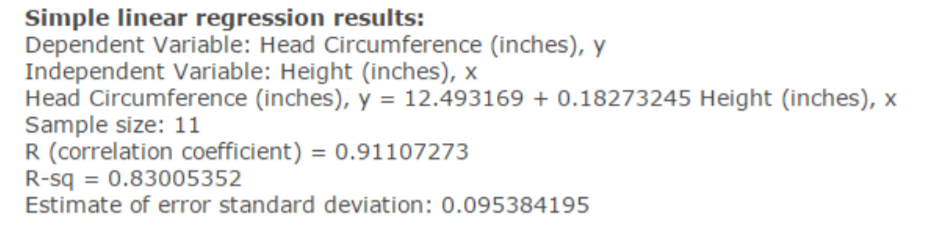# Simple linear regression results:Dependent Variable: Head Circumference (inches), yIndependent Variable: Height (inches), xHead Circumference (inches), y = 12.493169 + 0.18273245 Height (inches), xSample size: 11R (correlation coefficient) 0.91107273R-sq 0.83005352Estimate of error standard deviation: 0.095384195

Question
20 views

A pediatrician wants to determine the relationship that exists between a
child’s height, x, and head circumference, y. She randomly selects 11 children from her practice, measures their heights and head circumferences, and conducts the least-squares regression analysis with the simple linear model using StatCrunch. The output is given below:

(a) Write down the equation of the least-squares regression line treating height as the explanatory variable and head circumference as the response variable.

(b) Interpret the slope and y-intercept, if appropriate.

(c) Use the regression equation to predict the head circumference of a child who is 25 inches tall. Assume that the regression model is applicable.

(d) It is observed that one child who is 25 inches tall has a head circumference of 17.5 inches. Is the observed value above or below average among all children with heights of 25 inches?help_outlineImage TranscriptioncloseSimple linear regression results: Dependent Variable: Head Circumference (inches), y Independent Variable: Height (inches), x Head Circumference (inches), y = 12.493169 + 0.18273245 Height (inches), x Sample size: 11 R (correlation coefficient) 0.91107273 R-sq 0.83005352 Estimate of error standard deviation: 0.095384195 fullscreen
check_circle

Step 1

Note:

Hi there! Thank you for posting the question. As your question has more than 3 parts, we have solved the first 3 for you, according to our policy. If you need help with any other part, please re-post the question and mention the part you need help with.

Step 2

(a) Equation of the least-squares regression line:

The equation of the least-squares regression line is y-cap = β0 + β1x.

From the StatCrunch output, it is observed that the least-squares regression line treating height as the explanatory variable and head circumference as the response variable is,

Step 3

(b) Interpretation of slope and y-intercept:

In a regression line equation, β0 refers to the y-intercept and β1 refers to the slope of the line.

Here, the value of β0 is 12.49 (approx.) and the value of β1 is 0.18 (approx.)

Slope and y-intercept:

The value of slope refers that the change in value of y for one unit changes in x. The y-intercept is the value at which line crosses the y axis. That is, it is the value when x is 0.

Interpretation of slope:

The slope v...

### Want to see the full answer?

See Solution

#### Want to see this answer and more?

Solutions are written by subject experts who are available 24/7. Questions are typically answered within 1 hour.*

See Solution
*Response times may vary by subject and question.
Tagged in

### Statistics